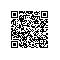# uva 639 - Don't Get Rooked

//暴力枚举2^16种解
//我们知道对于这个搜索的解空间树的解集最多有2的16次方种，那么复杂度就比较小，我么可以去枚举每一个解，然后找到其中的一个最优解。对于这道题而言，每一个位置的状态就是放与不放，转化为0 1思想（1表示放，0表示不放），我们可以用一个数组最大16位，存储从第一层到最后一层的最后一个，这样每个点的状态就被表示出来了，如何得到这个数组呢，我们从最大数开始，对于n*n的而言，最大数为2^(n*n) -1,我们用二进制的思想每一次把数和1做&运算，然后逆向存储到数组里面，做完把数右移一位即可省区前面许多没用的位数。
#include <iostream>
#include <cstdio>
#include <cstring>
#include <cstdlib>
#include <list>
#include <vector>
#include <stack>
#include <cmath>
#include <algorithm>
using namespace std;
const int MAXN = 4;

int n , cnt , pos , ans , flag;
bool  bite[MAXN*MAXN];//存储点的状态
char G[MAXN][MAXN];//存储输入的地图
int dir = {{-1,0},{0,1},{1,0},{0,-1}};//方向数组

//判断是否满足条件
//如果碰到'X'直接退出，碰到'.'且bite值为1那么直接返回0，否则返回1
int judge(int x , int y){
for(int i = x-1 ; i >= 0  ; i--){
if(bite[i*n+y] &&G[i][y] == '.')
return 0;
if(G[i][y] == 'X')
break;
}
for(int i = x+1 ; i < n  ; i++){
if(bite[i*n+y] &&G[i][y] == '.')
return 0;
if(G[i][y] == 'X')
break;
}
for(int i = y-1 ; i >= 0  ; i--){
if(bite[x*n+i] &&G[x][i] == '.')
return 0;
if(G[x][i] == 'X')
break;
}
for(int i = y+1 ; i < n  ; i++){
if(bite[x*n+i] &&G[x][i] == '.')
return 0;
if(G[x][i] == 'X')
break;
}
return 1;
}
//每次传入一个数进行解的计算
void solve(int num){
int i , j;
flag = 0;
pos = n*n - 1;//pos指向bite数组的下标
memset(bite , 0 , sizeof(bite));
while(pos >= 0){
bite[pos] = num & 1;//每一和1&运算
--pos;//向前移动
num >>= 1;//右移一位
}
//判断是否满足，对于bite数组是1的才判断
for(i = 0 ; i < n ; i++){
for(j = 0 ; j < n ; j++){
if(bite[i*n+j]){
if(G[i][j] == 'X')//如果是'X'而该点为1则可以直接返回说明该解不可能有
return;
if(G[i][j] == '.'){//如果是'.'判断
if(judge(i , j) == 0)
return;
}
}
}
}
//如果满足那么计算出数组中1的个数
for(i = 0 ; i < n*n ; i++){
if(bite[i])
++flag;
}
}

//主函数
int main(){
while(scanf("%d%*c" , &n) && n){
for(int i = 0 ; i < n ; i++){
for(int j = 0 ; j < n ; j++)
scanf("%c" , &G[i][j]);
getchar();
}
int m = n*n;
ans = 0;
cnt = pow(2 , m) - 1;//最大的数
while(cnt >= 0){//循环枚举解空间
solve(cnt);
--cnt;
ans = (ans > flag ? ans : flag);//求最大的ans
}
printf("%d\n" , ans);
}
return 0;
}


//我们可以用回溯来做，所谓的回溯就是在dfs上返回上一层时候多了个状态返回，其它都一样。
#include <iostream>
#include <cstdio>
#include <cstring>
#include <cstdlib>
#include <list>
#include <vector>
#include <stack>
#include <algorithm>
using namespace std;
const int MAXN = 5;

int n , ans , flag;
char G[MAXN][MAXN];
int vis[MAXN][MAXN]; //初始化'X'的位置为-1，其它为0

//判断是否满足条件

int judge(int x, int y) {
for (int i = x - 1; i >= 0; i--) {
if (vis[i][y] == 1)//如果是1说明不满足
return 0;
if (vis[i][y] == -1)//如果是'X'则退出
break;
}
for (int i = y - 1; i >= 0; i--) {
if (vis[x][i] == 1)
return 0;
if (vis[x][i] == -1)
break;
}
return 1;
}

//回溯搜索

void dfs(int i, int j, int max) {
if (max > ans)
ans = max;
while (i < n) {
if (j<n && G[i][j] == '.' && vis[i][j] == 0) {
flag = judge(i, j);
if (flag) {
vis[i][j] = 1;
dfs(i, j + 1, max + 1);
vis[i][j] = 0;
}
}
if (j >= n) {
++i;
j = 0;
}
else
++j;
}
}

//主函数

int main() {
//freopen("input.txt" , "r" , stdin);
while (scanf("%d%*c", &n) && n) {
memset(vis, 0, sizeof (vis));
for (int i = 0; i < n; i++) {
for (int j = 0; j < n; j++) {
scanf("%c", &G[i][j]);
if (G[i][j] == 'X') {
vis[i][j] = -1; //初始化vis数组
}
}
getchar();
}
ans = 0;
dfs(0, 0, 0); //调用函数（开始搜索子树）
printf("%d\n", ans);
}
return 0;
}使用钉钉扫一扫加入圈子
+ 订阅